# 1. Review from chapter 1 Behavior of fluids to Volume or Surface forces

 Lesson 3: Derivation of the Navier-Stokes equation in a non-rotating frame. 1. Review from chapter 1 - Behavior of fluids to Volume or Surface forces: Two types of forces act on Fluids: 1. Volume forces – Long rage forces capable of penetrating into the interior of the fluid and act on all elements. - Contact of source of force with material is not necessary. Examples of body forces in fluid systems: a. Gravity b. Electromagnetic Radiation c. Apparent forces due to coordinate system motion Surface forces – Short range forces that act only on a thin layer adjacent to the boundary of a fluid element. Surface forces are negligible unless there is direct mechanical between the interacting elements. Examples of surface forces in fluids: a. Pressure Fields b. Friction Provided the penetration depth of the short-range forces is small compared with the linear dimensions of the plane surface elements, the total force exerted across the surface element will be proportional to its area, . 2. Newton’s second law of motion – conservation of momentum Newton’s second law is expressed as the time derivative of momentum, (Momentum is not to be confused with the scalar pressure field, p) .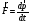Momentum is equal to the product of mass and velocity, . For the case of fluids, we prefer to deal with densities instead of volumes and the associated momentum density is and total momentum is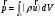and Newton’s second law then takes the form: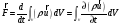(1) In index notation, Newton’s Second Law can be written as: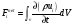. The net force in the above expression can be due to external forces such as those discussed in section (1) but also due to spatial variations of momentum across the fixed volume. These spatial variations lead to a momentum flux through the volume surface,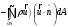. To get an idea of the effects of the momentum flux, imagine a solid box in space and think of all the molecules bombarding the surface of this box at a point in time. If the molecules on one surface have a larger net momentum on one side of the box than the other, then this will lead to a resulting force. Of course, our hypothetical fixed volume in fluid dynamics is not a solid but the principle remains the same in that the net momentum of the fluid along the surface of leads to a net force. Using Gauss’s theorem, the momentum flux can be expressed as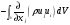and equation (1), in index form, is expressed as: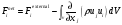. Returning to Newton’s Second Law,, we now get that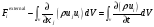. Solve for the external force by rearranging the above expression to isolate the external forces, and drop the external subscript: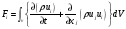Applying the product rule on both terms of the integrand and rearranging, we obtain: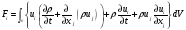The two terms on the left comprise the conservation of mass and are therefore equal to zero and so we obtain Newton’s second law for a fixed volume in the fluid: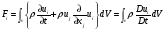(2) Deriving the Navier-stokes equation is now a matter of mathematically expressing the various external forces that are applied to this arbitrary fixed volume in space. The most formidable of these forces to describe are the net surface forces or stress fields. Share with your friends: